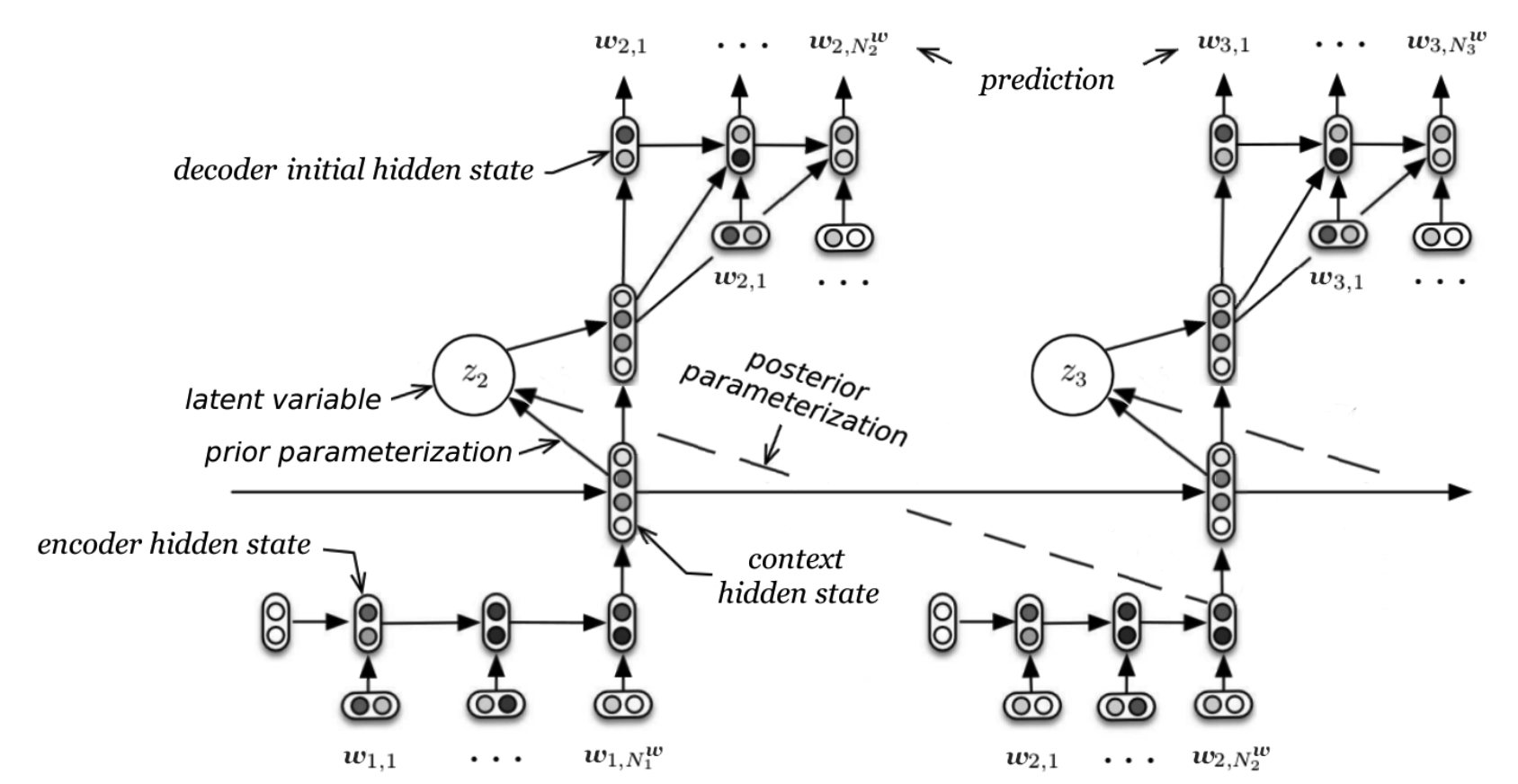# A Hierarchical Latent Variable Encoder-Decoder model for Generating Dialogues

## WHY?

Hierarchical recurrent encoder-decoder model(HRED) that aims to capture hierarchical structure of sequential data tends to fail because model is encouraged to capture only local structure and LSTM often has vanishing gradient effect.

## WHAT?Latent Variable Hierarchical Recurrent Encoder-Decoder(VHRED) tried to improve HRED by forcing to learn z with variational inference. Generative process output z from previous w, and inference process infer z through next w.

P_{\theta}(z_n|w_{<n}) = N(\mu_{prior}(w_{<n}), \Sigma_{prior}(w_{<n}))\\
P_{\theta}(w_n|z_n, w_{<n}) = \Pi_{m=1}^{M_n}P_{\theta}(w_{n,m}|z_n, w_{<n}, w_{n, <m})\\
log P_{\theta}(w_{<N}) \geq \Sigma_{n=1}^N - KL[Q_{\psi}(z_n|w_{\leq n})\|P_{\theta}(z_n|w_{<n})] + E_{Q_{\psi}(z_n|w_{\leq n})}[log P_{\theta}(w_n|z_n, w_{<n})]\\
Q_{\psi}(z_n|w_{\leq N}) = Q_{\psi}(z_n|w_{\leq n}) = N(\mu_{posterior}(w_{\leq n}), \Sigma_{posterior}(w_{\leq n}))

## So?

VHRED tends to perform better in Long context than LSTM and HRED.

## Critic

It is good to implement variational inference in sequential data but I’m not sure this significantly improved performance. This model can be used to make a good paragraph vector.

Powered by aiden